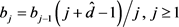﻿ 单位根过程与分整过程间变化变点的Sieve Bootstrap检验

# 单位根过程与分整过程间变化变点的Sieve Bootstrap检验Sieve Bootstrap Test for Changes between Unit Root Process and Fractional Integrated Processes

Abstract: This paper aimed to test change point from unit root process to fractional integrated process as well as fractional integrated process to unit process via a Dickey-Full ratio statistic. A Sieve Bootstrap method was proposed to determine the critical values. Simulations indicate that our proposed method can control the empirical size well both under the unit root and fractional integrated process null hypotheses, and gives satisfy empirical powers under two alternative hypotheses if the change point location does not too back. Furthermore, Dickey-Full ratio statistic has better performance when detecting those changes which from unit root process to fractional integrated process.

1. 引言

2. 模型与假设检验

${\left(1-L\right)}^{{d}_{0}}{X}_{t}={\epsilon }_{t},\text{ }\text{ }\text{ }\text{ }\text{ }t=1,2,\cdots ,n,$

${X}_{t}=\underset{j=0}{\overset{\infty }{\sum }}{w}_{j}\left({d}_{0}\right){\epsilon }_{t-j}.$

${X}_{t}~I\left(1\right)\left({d}_{0}=1\right),\text{\hspace{0.17em}}{X}_{t}~I\left({d}_{0}\right)\left(0<{d}_{0}<1,\text{ }\text{ }\text{ }且\text{ }\text{ }\text{ }{d}_{0}\ne 0\right)$

1) 序列 ${X}_{t}$$I\left(1\right)$$I\left({d}_{0}\right)$ 变化的持久性问题假设检验，即检验原假设

${H}_{0}^{1}:{X}_{t}~I\left(1\right),\text{ }\text{ }\text{ }\text{ }\text{ }\text{ }\text{ }\text{ }\text{ }\text{ }\text{ }\text{ }t=1,2,\cdots ,n.$

${H}_{1}^{1}:{X}_{t}~\left\{\begin{array}{l}I\left(1\right),\text{ }\text{ }\text{ }\text{ }\text{ }\text{ }\text{ }\text{ }\text{ }\text{ }\text{ }\text{ }\text{ }\text{ }\text{ }\text{ }\text{ }\text{ }\text{ }\text{ }\text{ }\text{ }\text{ }\text{ }t=1,\cdots ,{k}^{*},\\ I\left({d}_{0}\right),\text{ }\text{ }\text{ }\text{ }\text{ }\text{ }\text{ }\text{ }\text{ }\text{ }\text{ }\text{ }\text{ }\text{ }\text{ }\text{ }\text{ }\text{ }\text{ }t={k}^{\ast }+1,\cdots ,n.\end{array}$

2) 序列 ${X}_{t}$$I\left({d}_{0}\right)$$I\left(1\right)$ 变化的持久性问题假设检验，即检验原假设

${H}_{0}^{2}:{X}_{t}~I\left({d}_{0}\right),\text{ }\text{ }\text{ }\text{ }\text{ }\text{ }\text{ }\text{ }\text{ }\text{ }\text{ }\text{ }\text{ }t=1,2,\cdots ,n,$

${H}_{1}^{2}:{X}_{t}~\left\{\begin{array}{l}I\left({d}_{0}\right),\text{ }\text{ }\text{ }\text{ }\text{ }\text{ }\text{ }\text{ }\text{ }\text{ }\text{ }\text{ }\text{ }\text{ }\text{ }\text{ }\text{ }\text{ }\text{ }t=1,\cdots ,{k}^{*},\\ I\left(1\right),\text{ }\text{ }\text{ }\text{ }\text{ }\text{ }\text{ }\text{ }\text{ }\text{ }\text{ }\text{ }\text{ }\text{ }\text{ }\text{ }\text{ }\text{ }\text{ }\text{ }\text{ }\text{ }\text{ }\text{ }\text{ }t={k}^{\ast }+1,\cdots ,n.\end{array}$

${\Xi }_{n}\left(\tau \right)=|\frac{D{F}^{f}}{D{F}^{r}}|,$

3. Bootstrap方法

1) 估计 ${X}_{t}$ 的长记忆参数 ${d}_{0}$ ，并记估计值为 $\stackrel{^}{d}$

2) 对 ${X}_{t}$ 进行 $\stackrel{^}{d}$ 阶差分， ${\stackrel{^}{\epsilon }}_{t}={\left(1-L\right)}^{\stackrel{^}{d}}\left({X}_{t}-{X}_{t-1}\right),\text{\hspace{0.17em}}t=1,\cdots ,n$ 。其中 ${\left(1-L\right)}^{\stackrel{^}{d}}=\underset{j=0}{\overset{t}{\sum }}{\stackrel{^}{a}}_{j}{L}^{j},\text{\hspace{0.17em}}{a}_{0}=1$${a}_{j}={a}_{j-1}\left(j-\stackrel{^}{d}-1\right)/j,\text{\hspace{0.17em}}j\ge 1$

3) 对 ${\stackrel{^}{\epsilon }}_{1},\cdots ,{\stackrel{^}{\epsilon }}_{t}$ 进行重抽样，得到新的序列 ${\stackrel{^}{\epsilon }}_{ij}^{\ast },\cdots ,{\stackrel{^}{\epsilon }}_{nj}^{*}$

4) 生成Bootstrap样本 ${\stackrel{^}{Y}}_{tj}^{\ast }={\left(1-L\right)}^{-\stackrel{^}{d}}{\stackrel{^}{\epsilon }}_{tj}^{\ast }+{\stackrel{^}{z}}_{tj}$ ，其中 ${\left(1-L\right)}^{-\stackrel{^}{d}}=\underset{s=0}{\overset{t}{\sum }}{b}_{j}{L}^{j},\text{\hspace{0.17em}}{b}_{0}=1$${\stackrel{^}{z}}_{tj}$ 是独立同分布的标准正态分布随机序列。

5) 计算统计量 ${\Xi }_{n}\left({\stackrel{^}{Y}}_{tj}^{\ast }\right)$ ，重复步骤3~5 B次，取 ${\Xi }_{n}\left({\stackrel{^}{Y}}_{tj}^{\ast }\right)$$1-\alpha$ 分位数作为检验统计量在显著水平a

4. 数值模拟Table 1. Empirical sizes of Ξ n ( X ) (%)Table 2. Power values of Ξ n ( X ) (%)

5. 总结

 Hakkio, A.W. and Rush, M. (1991) Is the Budget Deficit Too Large? Econometrics Inquiry, 29, 429-445.

 Kim, J.Y. (2000) Detection of Change in Persistence of Linear Times Series. Journal of Econometrics, 95, 97-116.
https://doi.org/10.1016/S0304-4076(99)00031-7

 Kim, J.Y., Belaire Franch, J. and Badilli Amador, R. (2002) Corri-gendum to “Detection of Change in Persistence of Linear Times Series”. Journal of Econometrics, 109, 389-392.
https://doi.org/10.1016/S0304-4076(02)00087-8

 Perron, P. (1989) The Great Crash, the Oil Price Shock, and the Unit Root Hypothesis. Econometrica, 57, 1361-1401.
https://doi.org/10.2307/1913712

 Perron, P. (1990) Testing for a Unit Root in a Time Series with a Changing Mean. Journal of Business & Economic Statistics, 8, 153-162.

 Anindya, Banerjee, Lumsdaine, R. and Stock, J. (1992) Recursive and Sequential Tests of the Unit-Root and Trend-Break Hypotheses: Theory and International Evidence. Journal of Business & Economic Statistics, 10, 271-287.

 Leybourne, S.J., Kim, T.H. and Taylor, A.M.R. (2006) Regression-Based Tests for a Change in Persistence. Oxford Bulletin of Economics & Statistics, 68, 595-621.
https://doi.org/10.1111/j.1468-0084.2006.00179.x

 Hassler, U. and Scheithauer, J. (2011) Detecting Changes from Short to Long Memory. Statistical Paper, 52, 847-870.
https://doi.org/10.1007/s00362-009-0292-y

 Sibbertsen, P. and Kruse, R. Testing for a Break in Persistence under Long-Range Dependencies.

 Buhlmann, P. (1997) Sieve Bootstrap for Time Series. Bernoulli, 3, 123-148.
https://doi.org/10.2307/3318584

 Poskitt, D.S. (2008) Properties of the Sieve Bootstrap for Fractionally Integrated and Non-Invertible Processes. Journal of Time Series Analysis, 29, 224-250.
https://doi.org/10.1111/j.1467-9892.2007.00554.x

 Kapetanios, G. (2010) A Generalization of a Sieve Bootstrap In-variance Principle to Long Memory Processes. Quantitative and Qualitative Analysis in Social Sciences, 4, 19-40.

 Chen, Z., Tian, Z., Xiao, Q. and Xing, Y. (2014) Sieve Bootstrap Test for Variance Change in Long Memory Time Series. International Joint Conference on Applied Mathematics, 41, 61-73.

 Chen, Z., Tian, Z. and Xing, Y. (2016) Sieve Bootstrap Monitoring Per-sistence Change in Long Memory Process. Statistics & Its Interface, 9, 37-45.
https://doi.org/10.4310/SII.2016.v9.n1.a4

 Chen, Z., Xing, Y. and Li, F. (2016) Sieve Bootstrap Monitoring for Change from Short to Long Memory. Economics Letters, 140, 53-56.
https://doi.org/10.1016/j.econlet.2015.12.023

 Rambaccussing, D. (2015) A Test of the Long Memory Hypothesis Based on Self-Similarity. Journal of Time Series Econometrics, Sire Discussion Papers, 7.

 Robinson, P.M. (1995) Gaussian Semiparametric Estimation of Long Range Dependence. Annals of Statistics, 23, 1630-1661.
https://doi.org/10.1214/aos/1176324317

Top